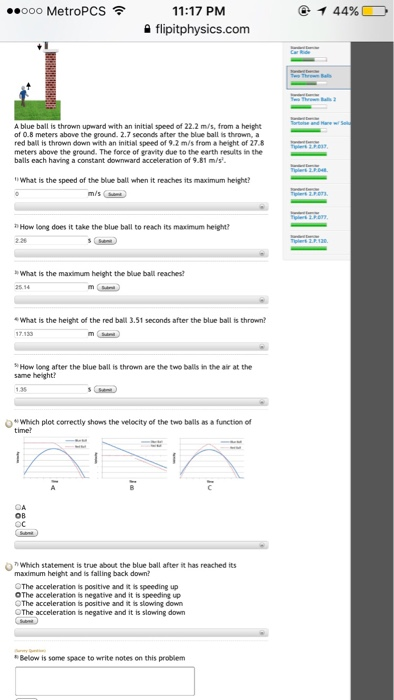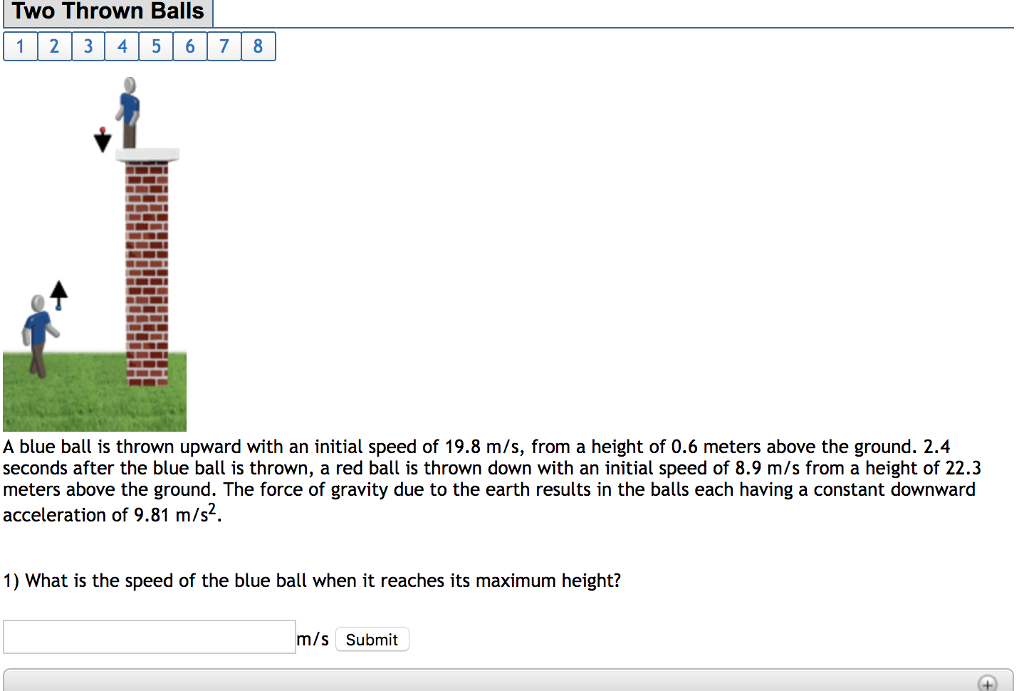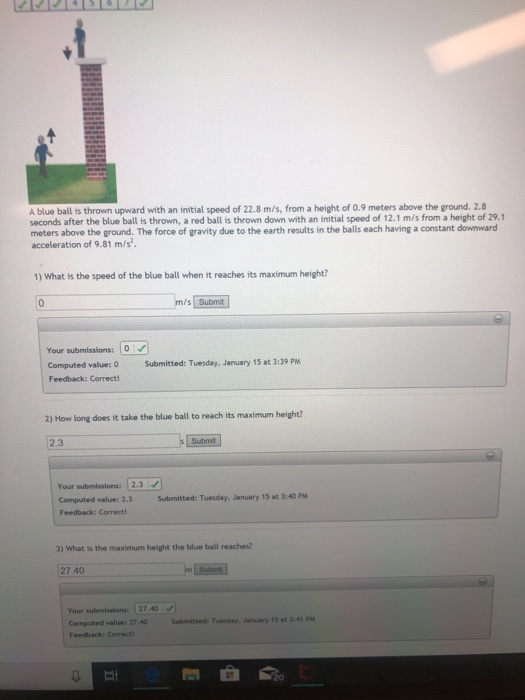Question

# A red ball is thrown down with an initial speed of 1.2 m/s from a height...

A red ball is thrown down with an initial speed of 1.2 m/s from a height of 25 meters above the ground. Then, 0.6 seconds after the red ball is thrown, a blue ball is thrown upward with an initial speed of 23.8 m/s, from a height of 0.8 meters above the ground. The force of gravity due to the earth results in the balls each having a constant downward acceleration of 9.81 m/s^2.

1)What is the speed of the red ball right before it hits the ground?

2)How long does it take the red ball to reach the ground?

3)What is the maximum height the blue ball reaches?

4) What is the height of the blue ball 1.8 seconds after the red ball is thrown?

5)How long after the red ball is thrown are the two balls in the air at the same height?

for red ball

initial velocity vr1 = 1.2 m/s

initial position yr1 = 0

for blue ball

initial velocity vb1 = 23.8 m/s

initial position yb1 = 0.8 m

---------------

1)

vr2^2 - vr1^2 = 2*ay*(yr2 - yr1)

vr2^2 - 1.2^2 = -2*9.81*(0-25)

vr2 = 22.2 m/s

===================

2)

yr2 - yr1 = vr1*t + (1/2)*ay*t^2

0 - 25 = 1.2*t - (1/2)*9.8*t^2

t = 2.38 s

===========================

3)

at maximum height final speed = 0

vb2 = 0

vb2^2 - vb1^2 = 28ay*(yb2 - yb1)

0^2 - 23.8^2 = -2*9.81*(yb2 - 0.8)

yb2 = 29.7 m

======================

4)

yb3 - yb1 = vb1*(1.8-0.6) + (1/2)*ay*(1.8-0.6)^2

yb3 - 0.8 = 23.8*(1.8-0.6) - (1/2)*9.81*(1.8-0.6)^2

yb3 = 22.3 m

=========================

5)

after time t the ball are at same height

for red ball

yr = yr1 + vr1*t + (1/2)*ay*t^2

for blue ball

yb = yb1 + vb1*(t-0.6) + 91/20*ay*(t-0.6)^2

yb = yr

25 + 1.2*t - (1/2)*9.81*t^2 = 0.8 + 23.8*(t-0.6) - (1/2)*9.81*(t-0.6)^2

t = 1.413 s

#### Earn Coins

Coins can be redeemed for fabulous gifts.

Similar Homework Help Questions
• ### A red ball is thrown down with an initial speed of 1.2 m/s from a height...

A red ball is thrown down with an initial speed of 1.2 m/s from a height of 26 meters above the ground. Then, 0.4 seconds after the red ball is thrown, a blue ball is thrown upward with an initial speed of 23.8 m/s, from a height of 1 meters above the ground. The force of gravity due to the earth results in the balls each having a constant downward acceleration of 9.81 m/s2. 1) What is the speed of...

• ### A blue ball is thrown upward with an initial speed of 24.1 m/s, from a height...

A blue ball is thrown upward with an initial speed of 24.1 m/s, from a height of 0.8 meters above the ground. 2.9 seconds after the blue ball is thrown, a red ball is thrown down with an initial speed of 10.4 m/s from a height of 31.4 meters above the ground. The force of gravity due to the earth results in the balls each having a constant downward acceleration of 9.81 m/s2. What is the height of the red...

• ### A blue ball is thrown upward with an initial speed of 23.9 m/s, from a height...

A blue ball is thrown upward with an initial speed of 23.9 m/s, from a height of 0.5 meters above the ground. 2.9 seconds after the blue ball is thrown, a red ball is thrown down with an initial speed of 7.5 m/s from a height of 31.5 meters above the ground. The force of gravity due to the earth results in the balls each having a constant downward acceleration of 9.81 m/s^2. How long after the blue ball is...

• ### A blue ball is thrown upward with an initial speed of 19.8 m/s, from a height of 0.5 meters above the ground

A blue ball is thrown upward with an initial speed of 19.8 m/s, from a height of 0.5 meters above the ground. 2.4 seconds after the blue ball is thrown, a red ball is thrown down with an initial speed of 7.9 m/s from a height of 22.5 meters above the ground. The force of gravity due to the earth results in the balls each having a constant downward acceleration of 9.81 m/s2. What is the maximum height the blue...

• ### A blue ball is thrown upward with an initial speed of 20 m/s, from a height...

A blue ball is thrown upward with an initial speed of 20 m/s, from a height of 0.8 meters above the ground. 2.4 seconds after the blue ball is thrown, a red ball is thrown down with an initial speed of 5.3 m/s from a height of 22.4 meters above the ground. The force of gravity due to the earth results in the balls each having a constant downward acceleration of 9.81 m/s2. 1) How long after the blue ball...

• ### Two throw A blue ball is thrown upward with an initial speed of 22.2 m/s, from...Two throw A blue ball is thrown upward with an initial speed of 22.2 m/s, from a height of 0.8 meters above the ground. 2.7 seconds after the blue ball is thrown, a red ball is thrown down with an initial speed of 9.2 m/s from a height of 27.8 meters above the ground. The force of grandly due to the earth results in the balls each having a constant downward acceleration of 9.81 m/s^2. What is the speed of...

• ### Two Thrown Balls 1 2 3 45678 A blue ball is thrown upward with an initial...Two Thrown Balls 1 2 3 45678 A blue ball is thrown upward with an initial speed of 19.8 m/s, from a height of 0.6 meters above the ground. 2.4 seconds after the blue ball is thrown, a red ball is thrown down with an initial speed of 8.9 m/s from a height of 22.3 meters above the ground. The force of gravity due to the earth results in the bals each having a constant downward acceleration of 9.81 m/s2....

• ### Please help with the last few parts A blue ball is thrown upward with an initial...Please help with the last few parts A blue ball is thrown upward with an initial speed of 22.8 m/s, from a height of 0.9 meters above the ground. 2.8 seconds after the blue ball is thrown, a red ball is thrown down with an initial speed of 12.1 m/s from a height of 29.1 meters above the ground. The force of gravity due to the earth results in the balls each having a constant downward acceleration of 9.81 m/s...

• ### A ball is thrown vertically upward with a speed of 23.7 m/s from a height of 2.0 m

A ball is thrown vertically upward with a speed of 23.7 m/s from a height of 2.0 m. How long does it take to reach its high- est point? The acceleration of gravity is 9.81 m/s2 2) How long does the ball take to hit the ground after it reaches its highest point?

• ### A ball is thrown upward from the ground with an initial speed of 16.6 m/s; at...

A ball is thrown upward from the ground with an initial speed of 16.6 m/s; at the same instant, another ball is dropped from a building 20 m high. After how long will the balls be at the same height above the ground?